# HSSlive: Plus One & Plus Two Notes & Solutions for Kerala State Board

## AP Board Class 7 Maths Chapter 10 Construction of Triangles Unit Exercise Textbook Solutions PDF: Download Andhra Pradesh Board STD 7th Maths Chapter 10 Construction of Triangles Unit Exercise Book AnswersAP Board Class 7 Maths Chapter 10 Construction of Triangles Unit Exercise Textbook Solutions PDF: Download Andhra Pradesh Board STD 7th Maths Chapter 10 Construction of Triangles Unit Exercise Book Answers

## Andhra Pradesh State Board Class 7th Maths Chapter 10 Construction of Triangles Unit Exercise Books Solutions

 Board AP Board Materials Textbook Solutions/Guide Format DOC/PDF Class 7th Subject Maths Chapters Maths Chapter 10 Construction of Triangles Unit Exercise Provider Hsslive

2. Click on the Andhra Pradesh Board Class 7th Maths Chapter 10 Construction of Triangles Unit Exercise Answers.
3. Look for your Andhra Pradesh Board STD 7th Maths Chapter 10 Construction of Triangles Unit Exercise Textbooks PDF.
4. Now download or read the Andhra Pradesh Board Class 7th Maths Chapter 10 Construction of Triangles Unit Exercise Textbook Solutions for PDF Free.

## AP Board Class 7th Maths Chapter 10 Construction of Triangles Unit Exercise Textbooks Solutions with Answer PDF Download

Find below the list of all AP Board Class 7th Maths Chapter 10 Construction of Triangles Unit Exercise Textbook Solutions for PDF’s for you to download and prepare for the upcoming exams:

Question 1.
Construct ∆PQR with measurements PQ = 5.8 cm, QR = 6.5 cm and PR = 4.5 cm.
Given measurements of ∆PQR are PQ = 5.8 cm, QR = 6.5 cm and PR = 4.5 cm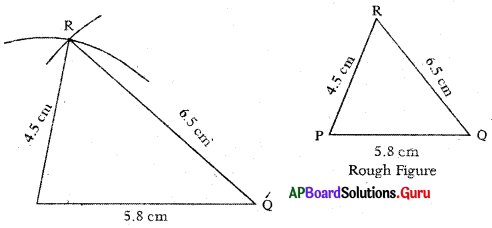Steps of Construction:

1. Draw a rough sketch of triangle arid label it with given measurements.
2. Draw a line segment with PQ = 5.8 cm.,
3. Draw ati arc with centre P and radius 4.5 cm.
4. Draw another arc with centre Q and radius 6.5 cm. to intersect the previous arc at R.
5. Join PR and QR.
Thus, required ∆PQR is constructed with the given measurements.

Question 2.
Construct an isosceles triangle LMN with measurements LM = LN = 6.5 cm and MN=8cm.
Given measurements of ∆LMN are LM = LN = 6.5 cm and MN = 8 cm.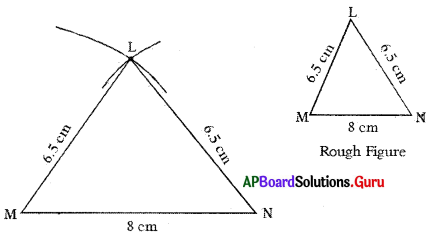Steps of Construction :

1. Draw a rough sketch of triangle and label it with given measurements.
2. Draw a line segment with MN = 8 cm.
3. Draw an arc with centre M and radius 6.5 cm.
4. Draw another arc with centre N and same radius (6.5 cm) to intersect the previous are at L
5. Join ML and NL.
Hence, required ∆LMN is constructed with the given measurements.

Question 3.
Construct ∆ABC with measurements ∠A = 60°, ∠B = 706 and AB = 7 cm.
Given measurements of ∆ABC are ∠A = 60°, ∠B = 70° and AB = 7 cm.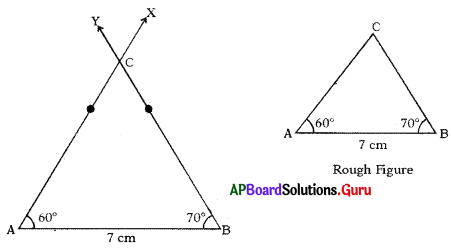Steps of Construction :

1. Draw a rough sketch of triangle and label it with given measurements.
2. Draw a line segment AB = 7 cm.
3. Draw a ray AX such that ∠BAX = 60°.
4. Draw another ray BY such that ∠ABY = 70°.
5. Name the intersecting point of AX and BY as C.
Hence, required ∆ABC is constructed with the given measurements.

Question 4.
Construct a right angled triangle XYZ in which ∠Y = 90°, XY = 5 cm and YZ = 7 cm.
Given measurements of ∆XYZ are ∠Y = 90°, XY = 5 cm and YZ = 7 Cm.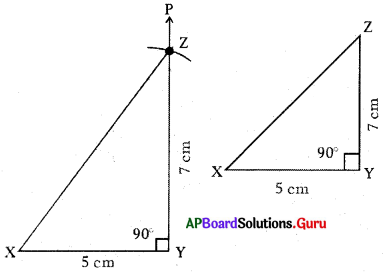Steps of Construction:

1. Draw a rough sketch of triangle and label it with given measurements.
2. Draw a line segment with XY = 5 cm.
3. Draw a ray YP such that ∠XYP = 90°
4. Draw an arc with centre Y and radius 7 cm to intersect YP at point Z.
5. Join XZ.
Hence, required ∆XYZ is constructed with the given measurements.

Question 5.
Construct an equilateral triangle DEF in which DE = EF = FD = 5 cm.
Given measurements of ∆DEF are DE = EF = FD = 5 cm.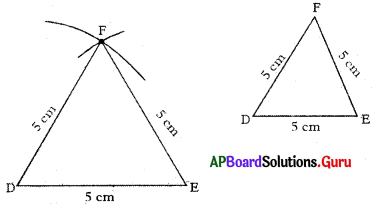Steps of Construction:

1. Draw a rough sketch of the triangle and label it with the given measurements.
2. Draw a line segment DE of length 5 cm.
3. Draw an arc with centre D and radius 5 cm.
4. Draw another arc with centre E and same radius (5 cm) to intersect the previous arc at F.
5. Join DF and EF.
Thus, required triangle ∆DEF is constructed with the given measurements.

Question 6.
Construct a triangle with non-included angle for the sides of ST and SU of lengths 6 v and 7 cm. respectively and ∠T = 80°.
Given measurements of ∆STU are ST = 6 cm, SU = 7 cm and ∠T = 80°.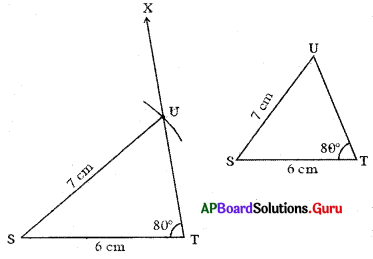Steps of Construction:

1. Draw a rough sketch of triangle and label it with given measurements.
2. Draw a line segment with ST = 6 cm.
3. Draw a ray TX such that ∠STX = 80°.
4. Draw an arc with centre S and radius 7 cm to intersect TX at point U.
5. Join SU.
Hence, required triangle ∆STU is constructed with the given measurements.

Question 7.
Can you construct ∆DEF with DE = 7 cm, EF = 14 cm and FD = 5 cm. ? If not give reasons. .
No, we can’t construct the triangle. Because the given sides of ∆DEF are DE = 7 cm, EF = 14 cm, FD = 5 cm.
In any triangle sum of any. two sides is always greater than the third side.
DE + FD = 7 + 5 = 12 cm < 14 cm
Sum of DE + FD < EF.
So, with the given measurements construction of ADEF is not possible

## Andhra Pradesh Board Class 7th Maths Chapter 10 Construction of Triangles Unit Exercise Textbooks for Exam Preparations

Andhra Pradesh Board Class 7th Maths Chapter 10 Construction of Triangles Unit Exercise Textbook Solutions can be of great help in your Andhra Pradesh Board Class 7th Maths Chapter 10 Construction of Triangles Unit Exercise exam preparation. The AP Board STD 7th Maths Chapter 10 Construction of Triangles Unit Exercise Textbooks study material, used with the English medium textbooks, can help you complete the entire Class 7th Maths Chapter 10 Construction of Triangles Unit Exercise Books State Board syllabus with maximum efficiency.

## FAQs Regarding Andhra Pradesh Board Class 7th Maths Chapter 10 Construction of Triangles Unit Exercise Textbook Solutions

#### Can we get a Andhra Pradesh State Board Book PDF for all Classes?

Yes you can get Andhra Pradesh Board Text Book PDF for all classes using the links provided in the above article.

## Important Terms

Andhra Pradesh Board Class 7th Maths Chapter 10 Construction of Triangles Unit Exercise, AP Board Class 7th Maths Chapter 10 Construction of Triangles Unit Exercise Textbooks, Andhra Pradesh State Board Class 7th Maths Chapter 10 Construction of Triangles Unit Exercise, Andhra Pradesh State Board Class 7th Maths Chapter 10 Construction of Triangles Unit Exercise Textbook solutions, AP Board Class 7th Maths Chapter 10 Construction of Triangles Unit Exercise Textbooks Solutions, Andhra Pradesh Board STD 7th Maths Chapter 10 Construction of Triangles Unit Exercise, AP Board STD 7th Maths Chapter 10 Construction of Triangles Unit Exercise Textbooks, Andhra Pradesh State Board STD 7th Maths Chapter 10 Construction of Triangles Unit Exercise, Andhra Pradesh State Board STD 7th Maths Chapter 10 Construction of Triangles Unit Exercise Textbook solutions, AP Board STD 7th Maths Chapter 10 Construction of Triangles Unit Exercise Textbooks Solutions,
Share: